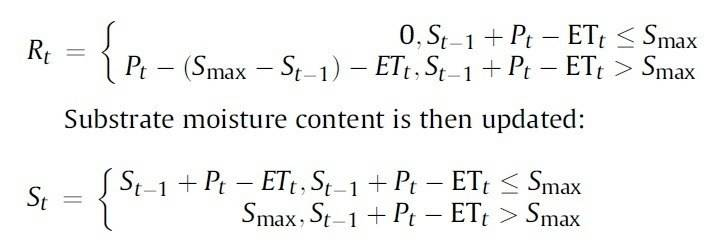# Help: MATLAB time step function

• MATLAB
TheodoreCT
Hi guys I'm new to MATLAB but now i need to use it for my study. I've tried to learn by myself to run it but unfortunately it failed, so I need some guidance on how to run following time step function in MATLAB

St = St-1 + Pt - ETt, St-1 + Pt - ETt ≤ Smax
= Smax, St-1 + Pt - ETt > Smax

then

Rt = 0, St-1 + Pt - ETt ≤ Smax
= Pt - (Smax - St-1) - ETt, St-1 + Pt - ETt > Smax

Pt = rainfall depth
ETt = evapotranspiration rate
S = Retained rainwater depth in substrate
Smax = maximum retention capacity
R = runoff
it is a hourly time step function, hourly rainfall data for a year will be inserted to the function
i would be really appreciated if anyone could help me.

Gold Member
I have a hard time understanding what your equations are trying to do,( although I think I can guess). Do commas separate equations? If so, what does "=Smax," at the beginning of line 2 mean? It may help if you add some comments and break up the lines into simpler parts. You will want to do that in the MATLAB code anyway. When code doesn't work, try to do calculations in baby steps. You can combine them later into more complicated equations.
 Oh, I see now that the comma means 'if' Maybe you should post the MATLAB code that you tried to run.

TheodoreCT
I have a hard time understanding what your equations are trying to do,( although I think I can guess). Do commas separate equations? If so, what does "=Smax," at the beginning of line 2 mean? It may help if you add some comments and break up the lines into simpler parts. You will want to do that in the MATLAB code anyway. When code doesn't work, try to do calculations in baby steps. You can combine them later into more complicated equations.
 Oh, I see now that the comma means 'if' Maybe you should post the MATLAB code that you tried to run.

thank you for your kind response, maybe you can refer to the pic below & the function actually looks like thisi don't know how to explain the commas (sorry i'm not good in explaining things) , but its similar to simple function like this
Rt = (R1, R2, R3...) where R1, R2, R3 is the Rt calculated at every hour.
In this case, Smax = 16mm, Rt and St equations are different before and after it reach Smax

Gold Member
Can you show what you tried to do for this so far? At the very least you should give more info about what is constant and what isn't, so that we can better help. Also show some sample data that will be fed in.

It should be rather straightforward to loop through your data and calculate these values once the problem is properly stated.

Gold Member
Ok. Here is something to get you started. I am at home and can't test this, so you will probably have to work on it to get it to run correctly. Also, you may have to change the indices of P and ET to get everything lined up correctly.

Code:
% find out how much data there is
[num_Pts, temp] = size(P);

% set initial values for t=1;
S(1) = 0;  % initial amount in the substrate
P(1) = 0;
ET(1) = 0;

% loop through the data and do the calculations
for t=(2:num_Pts)
% calculate total  for time t as though there is no limit
before_limiting = S(t-1) + P(t) - ET(t);

% if the limit is not exceeded, it is all retained in S and there is no runoff R
if before_limiting <= Smax
S(t) = before_limiting;
R(t) = 0;
else
% if the limit is exceeded, the max is retained in S and the rest is runoff
S(t) = Smax;
R(t) = before_limiting - Smax;
end
end